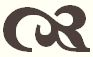#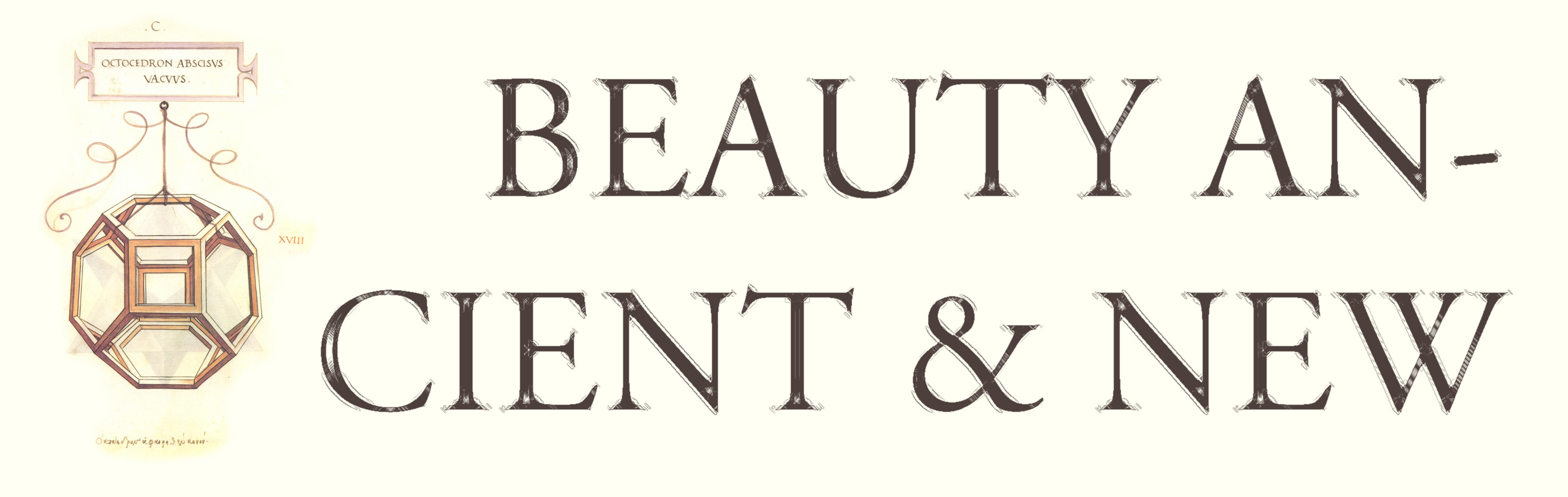Conic Sections

 Previous Contents Next

## Conic Graph Paper

A locus of points is a set of points satisfying some requirement. For instance, a circle with radius r and center O is the locus of points P such that OP = r. Or again, given a line segment AB, the perpendicular bisector of AB is the locus of points P such that AP = BP.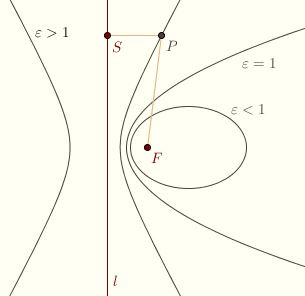Given a line l (the directrix), a point F (the focus), and a ratio ε > 0 (the eccentricity), the locus of points P such that the distance from P to F and the perpendicular distance from P to l are in the ratio ε is called a conic. If ε < 1, the conic is called an ellipse; if ε = 1, a parabola; if ε > 1, a hyperbola.

The line through the focus perpendicular to the directrix is called the axis of the conic. The points where the conic intersects the axis are called the vertices of the conic. The distance between the focus and the closest vertex is called the focal length of the conic.

If P is a point on the conic and S is the foot of the perpendicular from P to l, then the ratio PF/PS is equal to ε. We can see from the picture that the ellipse is a closed curve and that the hyperbola consists of two disconnected branches. The parabola is a kind of "boundary" case between the family of ellipses and the family of hyperbolas. Click here for animated version, which goes from ε = 0 to ε = ∞.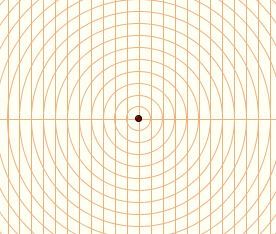Conics can be sketched using "graph paper" as shown. The focus lies at the center of a sequence of concentric circles. Starting with a line through the focus, a family of parallel lines is constructed so that the line spacing is the same as the circle spacing. The axis is the line through the focus perpendicular to every line in this family.

Begin by selecting one of the parallel lines as a directrix. Then select an intersection point on the axis between the focus and the directrix. This is our vertex.

For instance, we might select the line 12 units to the left of the focus, and the point on the axis 5 units from the focus. The eccentricity of the conic through this point would be 5/7; the focal length would be 5. Since 5/7 < 1, we know that the conic will be an ellipse. Any grid point on the ellipse satisfies the following: if p is the number of circle spaces to the focus and q is the number of line spaces to the directrix, then p/q = 5/7. So, for every 7 line spaces we move to the right, we move 5 circle spaces out. We have a pair of points on the 14th line from the directrix and the 10th circle from the focus, and another pair on the 21st line and the 15th circle, and another on the 28th line and the 20th circle, and a pair on the 35th line and the 25th circle, and a single point on the 42nd line and the 30th circle.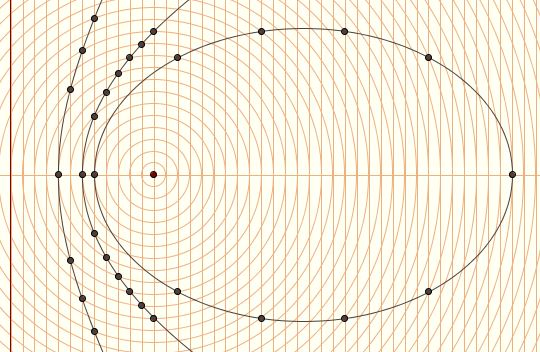It's a bit like we're graphing a line of slope 5/7. We view 5/7 as rise over run, where "run" refers to line spacing and "rise" to circle spacing. Also graphed here are a parabola and one branch of a hyperbola. The hyperbola crosses the axis 4 units from the directrix and 8 units from the focus, so its eccentricity is 8/4 = 2/1 = 2. The "rise" is 2 and the "run" is 1, so, for every 1 line space we move to the right, we move 2 circle spaces out. The other branch of the hyperbola crosses 12 spaces to the right of the directrix. Finally, the parabola of course has eccentricity 1, so for every line space we move to the right, we move out 1 circle space.

It's one thing to read this and another to do it yourself, so make sure to try it on paper. A print-ready sheet of graph paper is provided here for your convenience. If you take the directrix as the 12th line, as we did above, you should try graphing conics with eccentricity 1/3, 1/2, 5/7, 1, 7/5, 2, and 3. After you do that, try taking the directrix to be the 20th line, and graph conics with eccentricity 1/3, 3/7, 2/3, 1, 3/2, 7/3, and 3. In each case make sure to plot both branches of the hyperbola if possible.

Next: Conics are Connected### Mathematical Miscellany